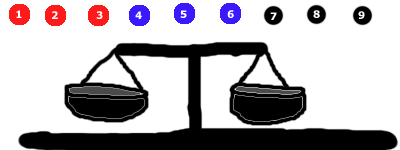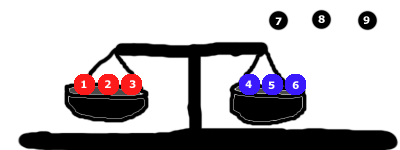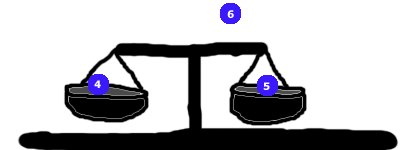# 9 marbles and a weight balance – which is the heaviest one?

So, you have 9 marbles and a balance. Or weight scale. Or weight balance. Or whatever you want to call it.

Essentially, this:My awesome drawing of a weight balance and 9 marbles

Because I draw like a 2 year old, some clarification as to what you see above. A real weight balance (or scale) looks slightly like the apparatus I drew above. You can put things in the little “buckets” and the scale will tip to the heavier side.

Yeah, I probably shouldn’t have even bothered with the picture. In any case, on to the riddle.

1) You have 9 marbles (as seen).

2) 8 of them are exactly the same weight. The remaining marble is slightly heavier than the others. You don’t know which is which.

3) You are allowed to use/set the scale TWICE in total. In other words, you get to take 2 measurements (or “comparisons”) here.

4) Each “bucket” has enough room for multiple marbles. As long as you have the same number of marbles in each side, the heavy marble has just enough weight to tip the scale to its side.

How do you find the heavy marble?

(scroll down slowly for tips and to eventually the answer!)

.

.

.

.

TIP #1: You won’t be putting all the marbles in for your first measurement (that wouldn’t work because we have an odd number!). You will be leaving at least 1 aside.

.

.

.

.

TIP #2: Clearly, you have to put an even number of marbles in each side for your first measurement. So either 1+1, 2+2, 3+3, or 4+4. Don’t forget, all the marbles are the same weight except for the 1 heavier one. That’s an important tidbit.

.

.

.

.

TIP #3: How many groups of marbles can you compare at once? If you said 2, think again!

.

.

.

.

TIP #4 (a big one, and part of the answer): Pretend you only had 3 marbles, but could only use the scale once. Could you figure out which of the 3 was the heavy one? (the answer is yes). How?

.

.

.

.

.

.

.

.We’ve separated the marbles into 3 groups. If you were stuck, the picture might help you with your first measurement.

.

.

.

.

Tip #6: Next image (solution to the 1st measurement) is below:

.

.

.

.Start by putting 3 marbles into each side. In this case, we leave the black ones out.

One of 3 things will happen:

• The red side may go down (in which case we know the red group contains the heaviest marble), or
• The blue side may go down (in which case we know the blue group contains the heaviest marble), or
• The sides will balance (in which case we know the black group must contain the heavy marble)

Either way we’ve narrowed it down from 9 possibilities to 3 on our first measurement.

Let’s keep going.

.

.

.

.

For argument’s sake, let’s pretend it was the blue marbles that had the heavy one in the measurement above. Let’s take our 2nd and final measurement.Similar to the first time around, we split these up evenly into 3 groups (3 groups of ONE this time).

One of three things will happen again:

• If #4 goes down, we know it is the heavy one
• If #5 goes down, we know it is the heavy one
• If the scale balances, we know #6 must be the heavy one!

Further thought (if that was too easy for you!):

To find the heavy marble took 2 measurements. How many measurements would we need if we had 27 marbles (26 being the same weight and 1 being heavier)?

What if we had 81 marbles (80 being even in weight and 1 being heavier)?

Well, this is the first brain teaser I’ve put up since 2006 (7 years… yikes!). But more should be coming soon. If you found this one interesting, you can hit up the “Riddles, Puzzles, Brain Teasers” section by clicking the category below or finding it in menu at the top of the site.

Sort by Oldest | Sort by Newest
I actually figured this one out in a couple minutes - usually I'm terrible at puzzles/riddles. Looking forward to the next one :D
good one
It's in with the final 2 images, but in case they're not loading:
1. We first put marbles in groups of 3. We want to see which group contains the heavy marble. To do this, put 3 marbles in each side (3 left, 3 right, 3 unused)
2. If the scale tips to a side, we know that group of 3 has the heavy marble. If it does not tip to a side (if it balances), we know the "unused" group of 3 contains the heavy marble. Keep the heavy group of 3 marbles and discard the rest.
3. Since we now have 3 marbles (1 of which is the heavy one), put 1 in each side (1 left, 1 right, 1 unused)
4. Set the scale again. If it tips to a side, we know that marble is the heavy one! If instead it balances, we know the one "unused" marble is the heavy one!
I have an alternative answer let me know what you think and if it has a higher probability of isolating the heavy one.

Remove one marble and put four and four one each side. If they are balanced the one you removed is the heaviest.

If they are unbalanced select the four from the heavier side. Remove one and isolate.

Replace that one with the one you originally removed because it has been ruled out. And do your second measure with two and two. If they are balanced the one you isolated is the heaviest.

If they are unbalanced on the side where you substituted the known marble. You can identify the heaviest.

If they are unbalanced on the 2 unknown you will have a 50/50 chance of choosing correctly. This solution provides 3 ways you could be absolutely sure.
The problem with your solution is that the "worst case" scenario would involve having to use the scale 3 times.

Given: Set A = (1, 2, 3, 4) and Set B = (5, 6, 7, 8), and Set C = (9)

On Measurement #1, we find that Set A is heavier than Set B
As per your solution, we discard Sets B and C and split Set A by isolating 1 of the marbles -- so now: Set A=(1, 2), Set B=(3), and Set C=(4).

Since you can't compare a set of 2 marbles against a set of 1 marble...we're left with just comparing B & C in Measurement #2. If either of those is heavier, great...the problem is solved. However, if they're balanced, then we then know that the heavy marble is in Set A....and that means making Measurement #3.